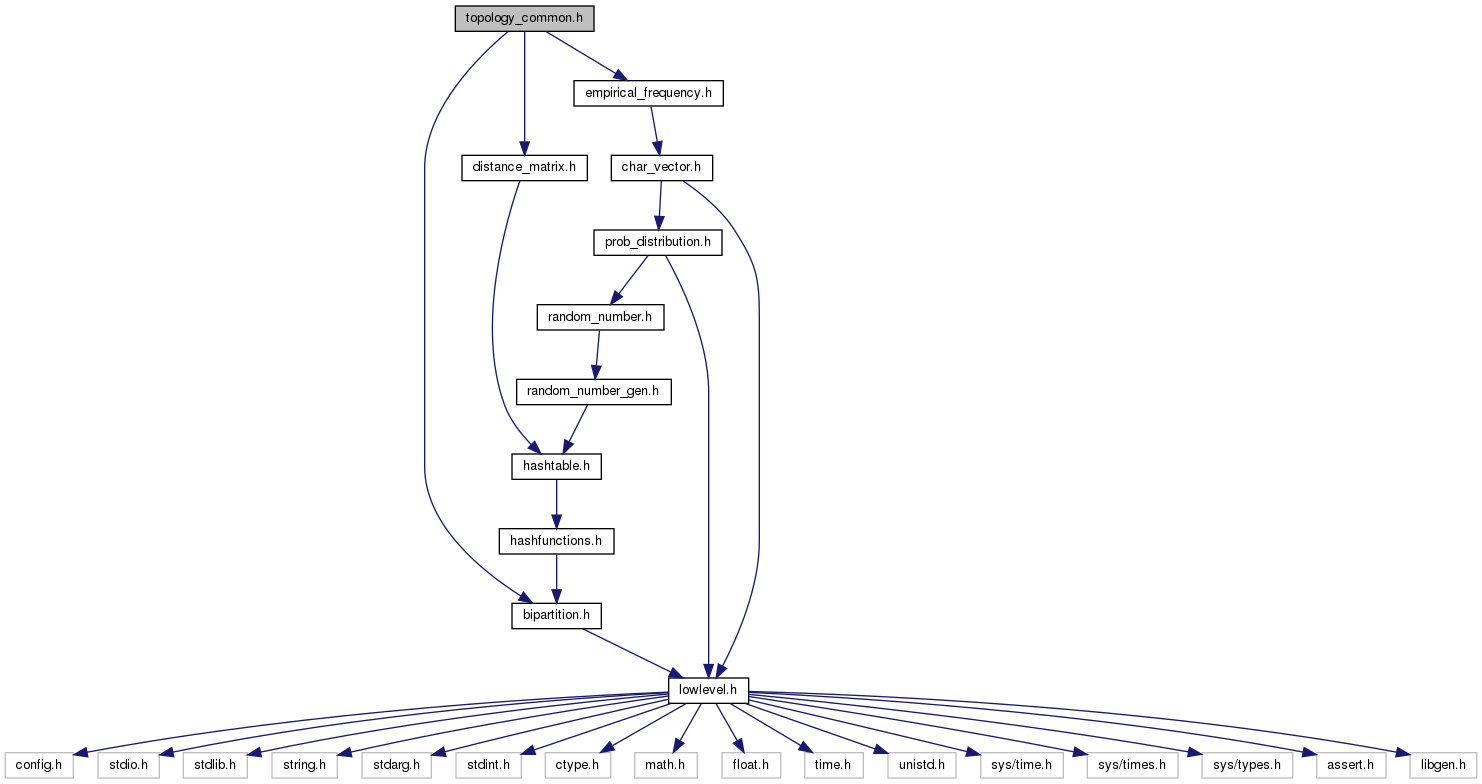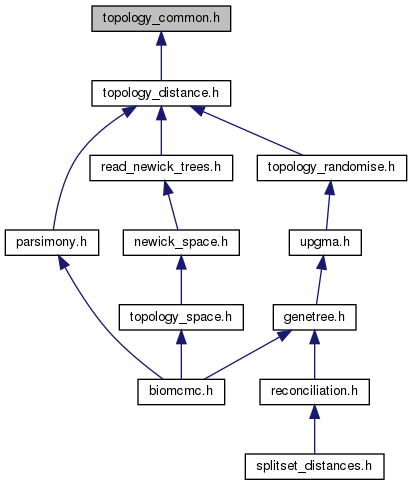biomcmc-lib  0.1 low level library for phylogenetic analysis
topology_common.h File Reference

General-purpose topology structures created from nexus_tree_struct (and low-level functions) More...

`#include "bipartition.h"`
`#include "distance_matrix.h"`
`#include "empirical_frequency.h"`
Include dependency graph for topology_common.h:This graph shows which files directly or indirectly include this file:Go to the source code of this file.

## Data Structures

struct  topol_node_struct
Information of a node (binary tree). More...

struct  topology_struct
Binary unrooted topology (rooted at leaf with ID zero) More...

## Typedefs

typedef struct topol_node_structtopol_node

typedef struct topology_structtopology

## Functions

topology new_topology (int nleaves)
Allocate space for new topology_struct.

void topology_malloc_blength (topology tree)
Allocate vector for branch lengths (3 vectors: mean, min and max values observed in topol_space collection)

void del_topology (topology topol)
Free space allocated by topology_struct.

void debug_topol (topology tree)

void copy_topology_from_topology (topology to_tree, topology from_tree)
Copy information from topology_struct. More...

void update_topology_sisters (topology tree)
Update pointers to topol_node_struct::sister.

void update_topology_traversal (topology tree)
Update topol_node::preorder, topol_node::postorder, topol_node::bipartition and order siblings by number of descendants.

bool topology_is_equal (topology t1, topology t2)
Compare two topologies based on bipartitions as clades (not on branch lengths)

bool topology_is_equal_unrooted (topology t1, topology t2, bool use_root_later)
Compare two topologies based on bipartitions neglecting root; boolean ask if split should be reverted to original orientation.

void reorder_topology_leaves (topology tree)
Reorder char_vector_struct; leaf node ids (and bipartitions) must then follow this order.

bool node1_is_child_of_node2 (topol_node node1, topol_node node2)
Boolean if node2 is on the path of node1 to the root.

char * topology_to_string_by_id (const topology tree, double *blen)
Print subtree in newick format to string using leaf IDs. More...

char * topology_to_string_create_name (const topology tree, double *blen)
Print subtree in newick format to string creating names (based on leaf IDs.) More...

char * topology_to_string_by_name (const topology tree, double *blen)
Print subtree in newick format to string using leaf names. More...

void graphviz_file_topology (FILE *fout, char *label, const topology tree)
Prints subtree in dot format to file. More...

void apply_spr_at_nodes (topology p, topol_node prune, topol_node regraft, bool update_done)
Apply one subtree prune-and-regraft (SPR branch swapping) operation at specified nodes. More...

void apply_spr_at_nodes_LCAprune (topology tree, topol_node prune, topol_node regraft, bool update_done)
Apply one SPR branch swapping at specified nodes when prune subtree is above prune node.

void apply_spr_at_nodes_notLCAprune (topology tree, topol_node prune, topol_node regraft, bool update_done)
Apply one SPR branch swapping at specified nodes when subtree to be pruned is below prune node. More...

void topology_undo_random_move (topology tree, bool update_done)
revert last SPR branch swapping

void clear_topology_flags (topology tree)
reset all d_done and u_done booleans to "true" (when rejecting a new state in MCMC)

void raise_topology_flags (topology tree)
reset all d_done and u_done booleans to "false" (when updating a model parameter with MTM)

void topology_reset_random_move (topology tree)
revert last SPR branch swapping and clear flags (reject last proposal, in MCMC)

int copy_topology_to_intvector_by_postorder (topology tree, int *ivec)
store ID of each node's parent (in postorder) into vector, returning number of stored nodes

int copy_intvector_to_topology_by_postorder (topology tree, int *ivec)
restore topological structure based on postordered ID vector, returning number of restored nodes

void copy_topology_to_intvector_by_id (topology tree, int *ivec)
store ID of each node's parent into vector

void copy_intvector_to_topology_by_id (topology tree, int *ivec)
restore topological structure based on ID vector

## Detailed Description

General-purpose topology structures created from nexus_tree_struct (and low-level functions)

The topology structure should actually be called "tree" since it has information about branch lengths, but these functions neglect branch lenght information. Here we have functions that create split bipartitions for edges (stored by nodes below the edge) and compare distinct topologies based on these bipartitions. We also have here the lowest-level function that apply an SPR on a topology (again, without caring about the branch length).

## ◆ copy_topology_from_topology()

 void copy_topology_from_topology ( topology to_tree, topology from_tree )

Copy information from topology_struct.

Since IDs do not change, this function only needs to update topol_node_struct::up, topol_node_struct::right, and topol_node_struct::left pointers and topol_node_struct::map_id from internal nodes; update of topol_node::sister is handled by function update_topology_sisters().

Parameters
 [in] from_tree original topology_struct [out] to_tree (previously allocated) copied topology_struct

## ◆ topology_to_string_by_id()

 char* topology_to_string_by_id ( const topology tree, double * blen )

Print subtree in newick format to string using leaf IDs.

Stores in string the tree in newick format, using leaf ID numbers (in practical applications needs a TRANSLATION nexus block). Memory allocation is handled by this function, but needs to be freed by the calling function.

Parameters
 [in] tree tree to be printed [in] blen vector with branch lengths (usually tree->blength)
Returns
a pointer to newly allocated string

## ◆ topology_to_string_create_name()

 char* topology_to_string_create_name ( const topology tree, double * blen )

Print subtree in newick format to string creating names (based on leaf IDs.)

Stores in string the tree in newick format, using newly-created names based on leaf ID numbers (useful for generating random trees that must be read by other programs.) Memory allocation is handled by this function, but needs to be freed by the calling function.

Parameters
 [in] tree tree to be printed [in] blen vector with branch lengths (usually tree->blength)
Returns
a pointer to newly allocated string

## ◆ topology_to_string_by_name()

 char* topology_to_string_by_name ( const topology tree, double * blen )

Print subtree in newick format to string using leaf names.

Stores in string the tree in newick format, preserving sequence names if available. Memory allocation is handled by this function, but needs to be freed by the calling function.

Parameters
 [in] tree tree to be printed [in] blen vector with branch lengths (usually tree->blength)
Returns
a pointer to newly allocated string

## ◆ graphviz_file_topology()

 void graphviz_file_topology ( FILE * fout, char * label, const topology tree )

Prints subtree in dot format to file.

Prints to file the tree in dot format (undirected graph). The dot format can be used with the graphviz suite of programs, and is not restricted to trees but can also handle arbitrary graph structures. Notice that we do not make use of the graphviz library, we simply create the text file graphviz programs take as input. Unfortunately, it is not helpful to print the nexus_tree_struct since the program works basically with the topology_struct. On the other hand it is easy to change this function to make it work with topology_struct.

Parameters
 [in,out] fout pointer to file handler where tree is to be printed; [in] label graph name or any other label; [in] tree topology_struct to be printed;

## ◆ apply_spr_at_nodes()

 void apply_spr_at_nodes ( topology p, topol_node prune, topol_node regraft, bool update_done )

Apply one subtree prune-and-regraft (SPR branch swapping) operation at specified nodes.

Each node is associated to one edge (the branch immediately above it), thus the location of the regraft node will impose the direction of pruning - the prune edge will always detach away from subtree containing regraft. The actual SPR move needs to handle two cases: prune node is in the path from regraft node to the root (prune node is least common ancestor between prune and regraft) and prune node is not in the path from regraft node to root (prune and regraft nodes share a distinct common ancestor). When prune node is the root, the first case implies in rerooting. Checking against illegal moves (prune==regraft, prune==regraft->up, etc) should be done previous to this function call. This function will call the corresponding lower-level one based on position of prune node. If you know the direction of pruning (rerooting, e.g.) you can call the other two functions directly.

Parameters
 [in,out] p topology over which to apply move [in] prune node to be pruned (detached). Direction determined by regraft [in] regraft node above which prune node will be reattached

## ◆ apply_spr_at_nodes_notLCAprune()

 void apply_spr_at_nodes_notLCAprune ( topology tree, topol_node prune, topol_node regraft, bool update_done )

Apply one SPR branch swapping at specified nodes when subtree to be pruned is below prune node.

prune is not lca: Detach the prune subtree and reinsert it just above the regraft node (regraft node may be root).

```*  Prune:
*
*  p.left\              /p.up.up                       p.left\                |p.up.up
*         \prune___p.up/                       ==>            \p_______prune  |
*         /            \                                      /               |
* p.right/              \p.up.left || p.up.right      p.right/                |p.up.left || p.up.right
*
* ```
```*  Regraft:
*
*  p.left\                |r.up        p.left\               /prune.up (=r.up.up)
*         \p_______prune  |      ==>          \p_______prune/
*         /               |                   /             \
* p.right/                |r          p.right/               \r
*
* ```